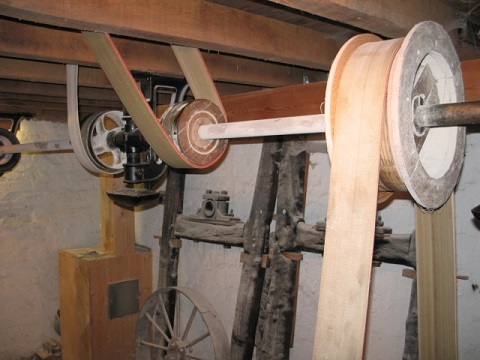﻿ Mechanics Map - Power in Rigid Body Systems

# Power in Rigid Body Systems

Related to the concepts of work and energy is the concept of power. At its core, power is the rate at which work is being done. Put into equation form, the power at any instant is defined as the derivative of work with respect to time.

 $P=\frac{dW}{dt}$

If we look at the average power over a set period of time, we can simply measure the work done and divide that by the time. Work for a rigid body is defined as the force times the distance the center of mass travels, plus the moment times angle of rotation (in radians) for our body.

 $P_{ave}=\frac{W}{t}=\frac{F*d+M*\Delta\theta}{t}$

Finally substituting in the definition of velocity (distance over time) and the definition of angular velocity (delta theta over time), we arrive at a third potential equation for power at a given instant.

 $P=F*v+M*\omega$

It should be noted that most devices will output power in either a translational fashion or in a rotational fashion, but rarely in both. In this case you would only need to account for the type of power being delivered by the device.

The common units of power are watts for metric, where one watt is defined as a joule per second, or a newton meter per second, and horsepower in the US Customary system where one horsepower is defined as 550 foot pounds per second.The drive shaft in this mill is used to transfer power from the input to the output. If we multiplied the toque in the shaft (in Newton meters) by the angular velocity (in radians per second) we would get the power being transmitted in watts (where a watt is a Newton meter per second). Image by Ian Petticrew CC-BY-SA 2.0

## Power and the Principle of Work and Energy

To use power as a term in work and energy problems, we will simply need to account for time as the new element in these problems. To do this, we can either multiply the average power by the time to get the total work done by a device, which then in turn will be equal to the change in energy.

 $P_{ave}*t=W=\Delta KE+\Delta PE$

Alternatively we could find the change in energy over the change in time, which will be equal to the average power exerted externally on the system.

 $P_{ave}=\frac{\Delta KE+\Delta PE}{\Delta t}$

## Worked Problems:

### Question 1:

The Ferris Wheel shown below can be approximated as a thin circular ring with a diameter of 96 meters and a mass of 20,000 kg. What is the power required from a motor in order to bring the Ferris wheel from rest to its operating speed of 0.5 rotations per minute over the course of 15 seconds?Image by Orlandowood365 CC-BY-SA 4.0

### Question 2:

A small off-road trailer can be approximated as a rectangular prism with a mass of 100kg supported by two wheels each approximated as thin circular discs with as mass of 25 kg and a diameter of 60 cm. Assuming the wheels roll without slipping, what is the power required to bring the trailer from rest to a speed of 80 kph over the course of 10 seconds?